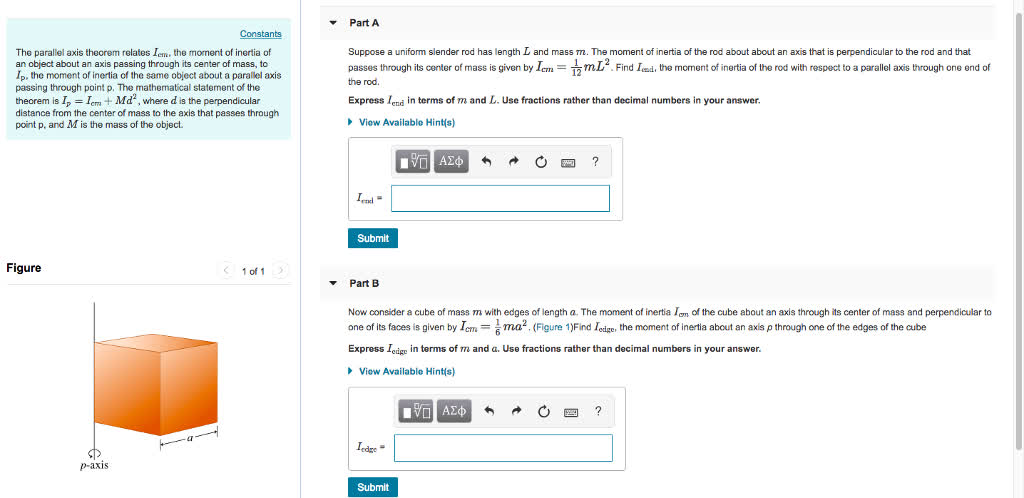# Part A Suppose a uniform slender rod has length L and mass m. The moment of inertia of the rod about about an axis that is perpendicular to the rod and thatFalak Kinney 2021-04-06 Answered

Part A Suppose a uniform slender rod has length L and mass m. The moment of inertia of the rod about about an axis that is perpendicular to the rod and thatYou can still ask an expert for help

• Questions are typically answered in as fast as 30 minutes

Solve your problem for the price of one coffee

• Math expert for every subject
• Pay only if we can solve itunessodopunsep
a) Using property given,
$I={I}_{cm}+M{d}^{2}$
Here, $d=\frac{L}{2}$
Hence,
$I=\frac{M{L}^{2}}{12}+\frac{M{L}^{2}}{4}=\frac{M{L}^{2}}{3}$
b) Using shift property of moment of inertia
$I=\frac{M{\alpha }^{2}}{6}+\frac{M{\alpha }^{2}}{2}=\frac{2M{\alpha }^{2}}{3}$